# Geometry - Grade 5 Math Educational Video (5.G.3)

##By Lumos Learning

Using the Lumos Study Programs, parents and educators can reinforce the classroom learning experience for children and help them succeed at school and on the standardized tests. Lumos books, dvd, eLearning and tutoring are used by leading schools, libraries and thousands of parents to supplement classroom learning and improve student achievement in the standardized tests.# Negative Exponents | MathHelp.com

##By MathHelp.com

In this example, we’re given the functions f(x) = 3x – 2 (read as “f of x equals…”) and g(x) = root x, and we’re asked to find the composite functions f(g(9)) (read as “f of g of 9”) and g(f(9). To find f(g(9)), we first find g(9). Since g(x) = root x, we can find g(9) by substituting a 9 in for the x in the function, to get g(9) = root 9, and the square root of 9 is 3, so g(9) = 3. Now, since g(9) = 3, f(g(9)) is the same thing as f(3), so our next step is to find f(3). And remember that f(x) = 3x – 2, so to find f(3), we substitute a 3 in for the x in the function, and we have f(3) = 3 times 3 minus 2. Notice that I always use parentheses when substituting a value into a function, in this case 3. Finally, 3 times 3 minus 2 simplifies to 9 minus 2, or 7, so f(3) = 7. Therefore, f(g(9)) = 7. Next, to find g(f(9), we first find f(9). Since f(x) = 3x - 2, we find f(9) by substituting a 9 in for the x in the function, to get f(9) = 3 times 9 minus 2, which simplifies to 27 – 2, or 25, so f(9) = 25. Now, since f(9) = 25, g(f(9)) is the same thing as g(25), so our next step is to find g(25). And remember that g(x) = root x, so to find g(25), we substitute a 25 in for the x in the function, to get g(25) = root 25. Finally, the square root of 25 is 5, so g(25) = 5. Therefore, g(f(9)) = 5. It’s important to recognize that# Angle Relationships 1 (7.G.5)

##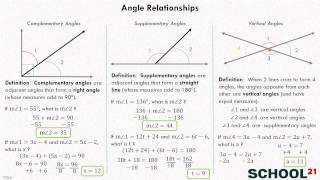By School 21# 8.G.4, 5~Transformation of a Line

##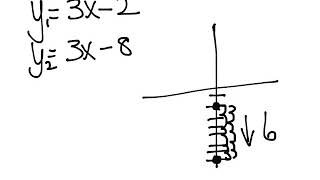By Elizabeth Knutson# 8.G.5 - Angle Intro and Vocabulary

##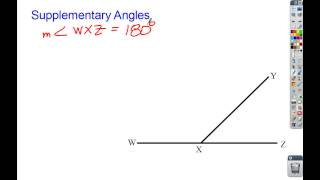By Clarkademy

Angle Intro and Vocabulary# Volume of Cylinder

##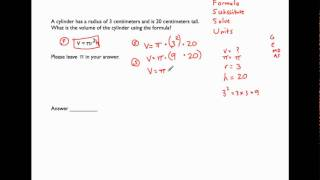By MathwithMrAlmeida

This video illustrates finding the volume of a cylinder, which is Common Core math standard 8.G.9. Watch from the beginning until 5:00 in the timecode ONLY for this standard.# Volume of Cylinder

##By MathwithMrAlmeida

This video illustrates finding the volume of a cylinder, which is Common Core math standard 8.G.9. Watch from the beginning until 5:00 in the timecode ONLY for this standard.# Triangle Angles and Similar Triangles 1 (8.G.5)

##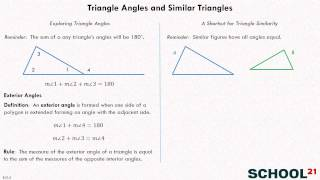By School 21# [5.G.3-1.0] Attributes & Categories - Common Core Standard

##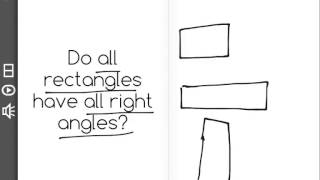By Front Row

Understand that attributes belonging to a category of two-dimensional figures also belong to all subcategories of that category. For example, all rectangles have four right angles and squares are rectangles, so all squares have four right angles.# [5.G.2-1.0] Points in Coordinate Plane - Common Core Standard

##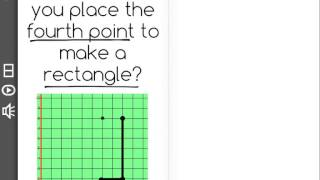By Freckle by Renaissance

Represent real world and mathematical problems by graphing points in the first quadrant of the coordinate plane. Front Row is a free, adaptive, Common Core aligned math program for teachers and students in kindergarten through eighth grade. Front Row allows students to practice math at their own pace - learning advanced concepts when they're ready and receiving remediation when they struggle. Front Row provides teachers with access to a detailed data dashboard and weekly email reports that show which standards are causing students difficulty, what small groups can be formed for interventions, and how their students are progressing in math.# [7.G.5-1.5] Angle Equations - Common Core Standard

##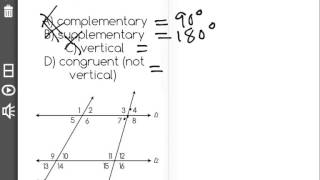By Freckle by Renaissance

Identify complementary, supplementary, vertical and adjacent angles Front Row is a free, adaptive, Common Core aligned math program for teachers and students in kindergarten through eighth grade. Front Row allows students to practice math at their own pace - learning advanced concepts when they're ready and receiving remediation when they struggle. Front Row provides teachers with access to a detailed data dashboard and weekly email reports that show which standards are causing students difficulty, what small groups can be formed for interventions, and how their students are progressing in math.# Coordinate plane word problems in the first quadrant

##By Khan Academy

Naomi needs some help counting the number of blocks she walks from home to school. We'll use the coordinate plane to graph it out first. Let's go.# Unknown angle algebra problems

##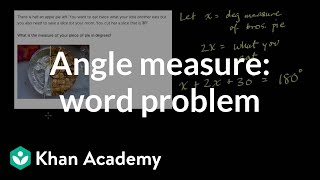By Khan Academy

Solve this word problem to find the measure of angles. In this example you'll split up a pie (don't forget to share!)# Unknown angle algebra problems

##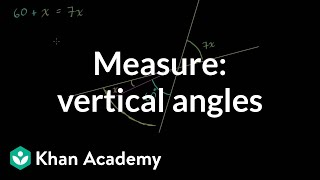By Khan Academy

Given two intersecting lines and the measure of vertical angles, watch as we solve to find the measure of the remaining angles.# Triangle angle example 1 | Angles and intersecting lines | Geometry | Khan Academy

##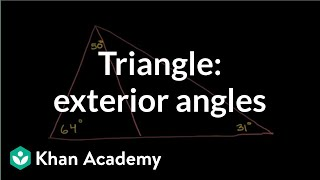By Khan Academy

Figuring out angles in a triangle. A little about exterior angles being the sum of the remote interior angles# SAT Math - Scholarly Unicorn - Lesson 4, Question 1

##By Dr.Steeve Warner

SAT Math - Scholarly Unicorn - Lesson 4, Question 1# Complementary and supplementary angles | Angles and intersecting lines | Geometry | Khan Academy

##By Khan Academy# Properties of shapes

##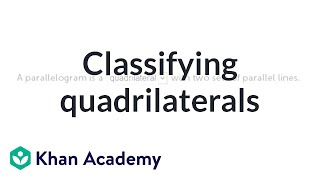By Khan Academy

Let's see how well you classify shapes by determining what properties match each kind of quadrilateral.# Composite Functions: f(g(x)) and g(f(x)) | MathHelp.com

##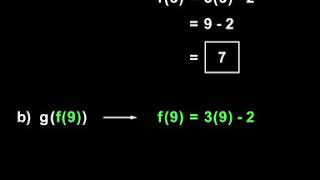By MathHelp.com

In this problem, we’re asked to add the given polynomials, then we’re asked to subtract the second polynomial from the first. In part a, to add the given polynomials, we simply add parentheses t^2 + 6t – 9 + parentheses t^2 + 7t - 3. Notice that I used parentheses around the polynomials. This is a good habit to get into, even though the parentheses will not affect the addition. Next, we simply add the like terms, t^2 + t^2 is 2t^2, 6t + 7t is 13t, and -9 - 3 is -12. So we have 2t^2 + 13t – 12. In part b, we’re asked to subtract the second polynomial from the first, so we have parentheses t^2 + 6t – 9 minus parentheses t^2 + 7t - 3. Notice that the second polynomial is subtracted from the first. And again, notice that we use parentheses around each polynomial. Now, it’s important to understand that the minus sign outside the second set of parentheses can be thought of as a negative 1, so we need to distribute the -1 through each of the terms in the second set of parentheses. So, after rewriting our first polynomial, t^2 + 6t – 9, we have -1 times t^2, or –t^2, -1 times positive 7t, which is -7t, and -1 times -3, which is positive 3. Now, we combine like terms. t^2 – t^2 cancels out, positive 6t minus 7t is -1t, or –t, and -9 + 3 is -6. So we have –t – 6. Makes sure to distribute the negative 1 through the parentheses when subtracting the second polynomial from the first.# Vertical angles

##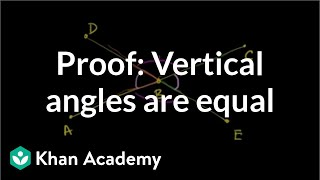By Khan Academy

Proving that vertical angles are equal